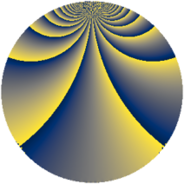# Properties

 Label 324.3.oLevel $324$ Weight $3$ Character orbit 324.o Rep. character $\chi_{324}(5,\cdot)$ Character field $\Q(\zeta_{54})$ Dimension $324$ Newform subspaces $1$ Sturm bound $162$ Trace bound $0$

# Related objects

## Defining parameters

 Level: $$N$$ $$=$$ $$324 = 2^{2} \cdot 3^{4}$$ Weight: $$k$$ $$=$$ $$3$$ Character orbit: $$[\chi]$$ $$=$$ 324.o (of order $$54$$ and degree $$18$$) Character conductor: $$\operatorname{cond}(\chi)$$ $$=$$ $$81$$ Character field: $$\Q(\zeta_{54})$$ Newform subspaces: $$1$$ Sturm bound: $$162$$ Trace bound: $$0$$

## Dimensions

The following table gives the dimensions of various subspaces of $$M_{3}(324, [\chi])$$.

Total New Old
Modular forms 1998 324 1674
Cusp forms 1890 324 1566
Eisenstein series 108 0 108

## Trace form

 $$324 q + O(q^{10})$$ $$324 q - 135 q^{21} - 81 q^{23} + 27 q^{27} + 81 q^{29} + 189 q^{33} + 243 q^{35} + 216 q^{41} + 432 q^{45} + 324 q^{47} + 126 q^{51} - 216 q^{57} - 378 q^{59} - 540 q^{63} - 108 q^{65} - 351 q^{67} + 504 q^{69} + 648 q^{71} + 450 q^{75} + 432 q^{77} - 54 q^{79} - 72 q^{81} - 216 q^{83} + 270 q^{85} - 1008 q^{87} - 648 q^{89} - 684 q^{93} - 432 q^{95} + 459 q^{97} - 252 q^{99} + O(q^{100})$$

## Decomposition of $$S_{3}^{\mathrm{new}}(324, [\chi])$$ into newform subspaces

Label Dim $A$ Field CM Traces $q$-expansion
$a_{2}$ $a_{3}$ $a_{5}$ $a_{7}$
324.3.o.a $324$ $8.828$ None $$0$$ $$0$$ $$0$$ $$0$$

## Decomposition of $$S_{3}^{\mathrm{old}}(324, [\chi])$$ into lower level spaces

$$S_{3}^{\mathrm{old}}(324, [\chi]) \cong$$ $$S_{3}^{\mathrm{new}}(81, [\chi])$$$$^{\oplus 3}$$$$\oplus$$$$S_{3}^{\mathrm{new}}(162, [\chi])$$$$^{\oplus 2}$$